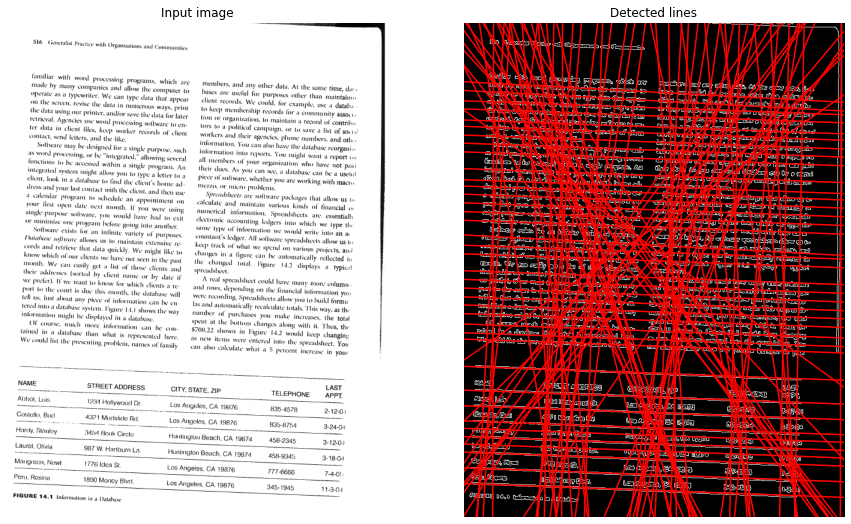# Skew Detection and Correction of Document images using Hough Transform

In this post I will be talking about how we can use Hough Transform to detect and correct Skewness of a document image. There have been many research papers published around this problem and it keeps getting published even today on various journals mainly because its still largely an unsolved problem. I had previously written about Skew correction using Horizontal Projections here which used the Horizontal projection of an image to indetify the skew angle, The problem with deskewing using Projections is that it fails in many cases where the text may have too many spaces and when the document has less text. This made me look for a better solution and I came across Hough Transform based skew detection technique which is has a better accuracy.

Hough transform is a feature extraction technique that converts an image from Cartesian to polar coordinates which is how it got “transform” in its name. It can be used to detect lines or a set of collinear points on the image. If you are new to Hough Transform I would recommend you take a look at the video below which is one of the best one on the internet on this topic.

The basic idea is:

• Convert the image to grayscale
• Apple Canny or Sobel filter
• Find Hough lines between 0.1 to 180 degree angle.
• Round the angles from line peaks to 2 decimal places.
• Find the angle with the highest occurrence.
• Rotate the image with that angle

Here is a sample image which is skewed.

After finding the Hough Lines

As you can see, we have detected a decent number of lines connecting our words. And all we have to do now is to find the orientation of the lines which connect the words.

The code to generate the Hough lines is as below.

``````import numpy as np
from skimage.transform import hough_line, hough_line_peaks
from skimage.transform import rotate
from skimage.feature import canny
from skimage.color import rgb2gray
import matplotlib.pyplot as plt
from scipy.stats import mode

edges = canny(image)
# Classic straight-line Hough transform
h, theta, d = hough_line(edges, theta=tested_angles)

# Generating figure 1
fig, axes = plt.subplots(1, 2, figsize=(15, 16))
ax = axes.ravel()

ax.imshow(image, cmap="gray")
ax.set_title('Input image')
ax.set_axis_off()

ax.imshow(edges, cmap="gray")
origin = np.array((0, image.shape))

for _, angle, dist in zip(*hough_line_peaks(h, theta, d)):
y0, y1 = (dist - origin * np.cos(angle)) / np.sin(angle)
ax.plot(origin, (y0, y1), '-r')

ax.set_xlim(origin)
ax.set_ylim((edges.shape, 0))
ax.set_axis_off()
ax.set_title('Detected lines')``````

Hough line method also gives us the angle made by the line with the origin as shown below.

As you might have guessed by now, we only need to isolate these potentially horizontal lines to get our skew angle. To do that, I am looking at the most commonly occurring angle and will rotate my image. The below method gives the skew angle.

``````def skew_angle_hough_transform(image):
# convert to edges
edges = canny(image)
# Classic straight-line Hough transform between 0.1 - 180 degrees.
h, theta, d = hough_line(edges, theta=tested_angles)

# find line peaks and angles
accum, angles, dists = hough_line_peaks(h, theta, d)

# round the angles to 2 decimal places and find the most common angle.
most_common_angle = mode(np.around(angles, decimals=2))

# convert the angle to degree for rotation.
return skew_angle``````

Here is the final output of Skew correction using Hough Transform. The angle of rotation is identified as: 2.24620502 degree.

Another important thing to note here is that, if the angle is greater than 90 degree which was the case in my sample, the image is titled clockwise and if it is its less than 90 degrees, its tilted anti-clockwise. In both cases we need to subtract the identified angle by 90 to get the rotation angle we need.

Here is the full notebook for your reference:

https://jovian.ml/viewer?url=https://github.com/muthuspark/ml_research/blob/master/Skew%20angle%20detection%20and%20correction%20using%20Hough%20Transform.ipynb

0 0 vote
Article Rating
Subscribe
Notify of